Published: 21 October 2017

# Study on dynamic characteristics of double cylinder double acting bilge pump transmission

Jiangmin Xia1
Zhuying Li2
Zhenhai Zhang3
Guodong Wei4
1, 2Department of Naval Architect Engineering, Naval University of Engineering, Wuhan, Hubei Province, China
3, 4College of Power Engineering, Naval University of Engineering, Wuhan, Hubei Province, China
Corresponding Author:
Jiangmin Xia
Views 53

#### Abstract

In view of the complex structure of the ship bilge pump, the excitation source in the process of work is difficult to accurately determine the fault condition of the bilge pump through the vibration test data. Based on the theory of multi - body dynamics, rotor dynamics and electrical mechanics, the kinematics and kinetic equations of the bilge pump drive are established, and the dynamics, kinematics and vibration characteristics of bottom Pump drive analysis of the cabin is carried out. The frequency of the excitation force corresponding to the transmission mechanism is deduced, and the frequency of the excitation force of the main transmission is calculated.

## 1. Introduction

Bilge pump is an important device to maintain the vitality of the ship, vibration abnormalities will seriously affect the stealth performance of ships . It is helpful to judge the performance status of the equipment in time and to accurately locate the faulty parts of the vibration and noise spectrum of the normal and abnormal state, and the main factors that affect the vibration and noise performance of the pump .

At present, the main technical problem is that the vibration characteristics of the bilge pump are not accurate, and it is difficult to accurately judge the technical status of the equipment. It is necessary to study the kinematics, dynamics and excitation characteristics of the equipment itself, including the motor, rolling bearing and the centrifugal force generated by the rotation of the crankshaft, by analyzing the characteristic frequency and the fault condition of the components of the equipment by the characteristic frequency. And then to establish the dynamic model and numerical calculation model of the mechanical parts of the bilge pump mechanical system. The research results can be used as the important basis for the monitoring of the bilge pump state and the vibration and noise control during the whole life period .

## 2. Double cylinder double acting bilge pump structure

A kind of bilge pump transmission system diagram shown in Fig. 1, the prime mover armature through the bearing installed in the motor body to drive the worm rotation, driven by the worm gear rotation, and the worm wheel and crankshaft meshing drive crankshaft rotation. The excitation source of the device itself is the centrifugal force of the motor, rolling bearing, gear transmission, piston inertia force, piston imbalance leading to crankshaft rotation .

### 2.1. Research on mathematical model of bilge pump drive

The pump transmission structure is divided into four parts: armature, crankshaft, connecting rod and piston. The mechanical model and equation of each part are established according to the interconnection of each part [5-7]. The armature rotor is simplified as a multi-stage continuous mass ladder axis, the establishment of armature structure model shown in Fig. 2. The reference base and the conjoined base are established based on the center of the bearing of the lower end of the motor. It is written as ${\stackrel{\to }{e}}^{r}=\left({\stackrel{\to }{x}}^{r},{\stackrel{\to }{y}}^{r}{,\stackrel{\to }{z}}^{r}\right)$ and ${\stackrel{\to }{e}}^{b}=\left({\stackrel{\to }{x}}^{b}{,\stackrel{\to }{y}}^{b}{,\stackrel{\to }{z}}^{b}\right)$. $\stackrel{\to }{{q}_{1}}$ of the armature coil in the magnetic field, the inertia force$\stackrel{\to }{{q}_{2}}$ caused by the rotor center of mass from the rotating shaft; the binding force includes the restraining force of the lower bearing to the shaft ${\stackrel{\to }{F}}_{O}=\left({\stackrel{\to }{F}}_{ox}{,\stackrel{\to }{F}}_{oy}{,\stackrel{\to }{F}}_{oz}\right)$ and ${\stackrel{\to }{F}}_{B}=\left({\stackrel{\to }{F}}_{Bx},{\stackrel{\to }{F}}_{By}{,\stackrel{\to }{F}}_{Bz}\right)$ .

Fig. 1The transmission system diagram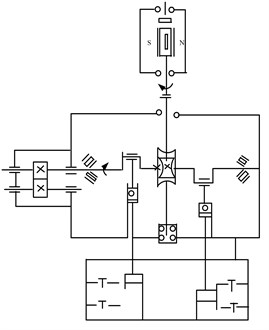Fig. 2The simplified model of armature rotor system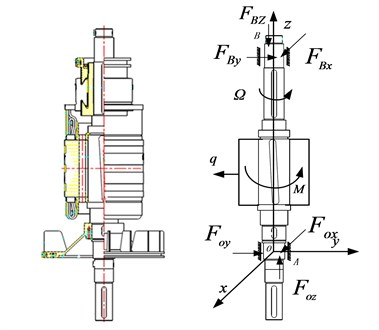The armature rotor transmits the interference force to the body through the bearing at both ends. Therefore, the analysis of the dynamic characteristics of the armature rotor is to determine the binding force ${\stackrel{\to }{F}}_{O}$ and ${\stackrel{\to }{F}}_{B}$. The differential equations of motion of the armature rotor are established by using the Timoshenko beam – axis model under the influence of the moment of inertia of the rotor and the shear deformation of the shaft on the dynamic characteristics of the motor armature. The motor armature rotor and its output shaft is approximately divided into five sections, namely the lower end of the shaft to the lower bearing section, the lower bearing to the lower end surface of the armature rotor, the armature rotor, the armature rotor upper surface to the upper bearing section, the upper end of the shaft is numbered $j=$ 1, 2, 3, 4, 5.

The deformation curves of the neutral axis are $x\left(z,t\right)$, $y\left(z,t\right)$, the cross section is ${\theta }_{x}\left(z,t\right)$, ${\theta }_{y}\left(z,t\right)$. To express in a plural number:

1
$w=x+iy，\theta ={\theta }_{x}+i{\theta }_{y}.$

The external force and shear stress $Q$ are also expressed in the plural form:

2
$M={M}_{x}+i{M}_{y},q={q}_{x}+i{q}_{y},Q={Q}_{x}+i{Q}_{y}.$

Use Timoshenko beam – axis model, the first – order differential equation of the axis is:

3
$\frac{{\partial }^{4}{w}_{1}}{\partial {z}^{4}}-\frac{\rho }{E}\left(1+\frac{E}{\mu G}\right)\frac{{\partial }^{4}{w}_{1}}{\partial {z}^{2}\partial {t}^{2}}+\frac{\mathrm{i}\mathrm{\rho }\mathrm{\Omega }}{E}\frac{{\partial }^{3}{w}_{1}}{\partial {z}^{2}\partial t}+\frac{\rho {A}_{i}}{EI}\frac{{\partial }^{2}{w}_{1}}{\partial {t}^{2}}-\frac{i{\rho }^{2}\mathrm{\Omega }}{E\mu G},$

where $\rho$ is the axis density, $E$ is the axial modulus, $G$ is the axial shear modulus, $\mu$ is the shear deformation coefficient, and $\mathrm{\Omega }$ is the axis rotation angle. The lower boundary condition is:

4
${\theta }_{1}-\frac{\partial {w}_{1}}{\partial z}=0,\frac{\partial {\theta }_{1}}{\partial z}=0.$

Since the lower bearing is simplified to elastic support and its stiffness is ${k}_{A}$, the boundary condition at the junction is:

5
$\left\{\begin{array}{l}\mu G{A}_{1}\left[{\theta }_{1}\left({l}_{1}\right)-\frac{\partial {w}_{1}\left({l}_{1}\right)}{\partial z}\right]-\mu G{A}_{2}\left[{\theta }_{2}\left(0\right)-\frac{\partial {w}_{2}\left(0\right)}{\partial z}\right]-{k}_{A}{w}_{2}\left(0\right)=0,\\ E{I}_{2}\frac{\partial {\theta }_{2}\left(0\right)}{\partial z}-E{I}_{1}\frac{\partial {\theta }_{1}\left({l}_{1}\right)}{\partial z}=0,\\ {w}_{1}\left({l}_{1}\right)={w}_{2}\left(0\right)=w,\\ {\theta }_{1}\left({l}_{1}\right)={\theta }_{2}\left(0\right),\end{array}\right\$

where ${A}_{1}$and ${A}_{2}$ are the cross-sectional areas of the first and second segments.

The armature is simplified as a clustering parameter model. As shown in Fig. 3, the translational displacement of the armature centroid $C$ is ${x}_{c}$, ${y}_{c}$, and the deviation angle around the centroid $c$ is ${\theta }_{x}$, ${\theta }_{y}$. The axial center-of-mass displacement of the both ends of the armature is ${x}_{1}$, ${y}_{1}$ and ${x}_{2}$, ${y}_{2}$, respectively:

6
${w}_{2}\left({l}_{2},t\right)={x}_{1}+i{y}_{1},{w}_{4}\left(0,t\right)={x}_{2}+i{y}_{2}.$

Fig. 3The armature rotor model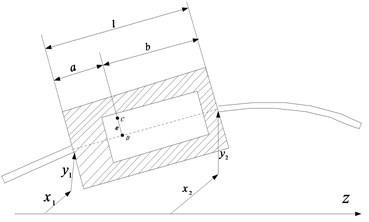Fig. 4The crank – slider body composition diagram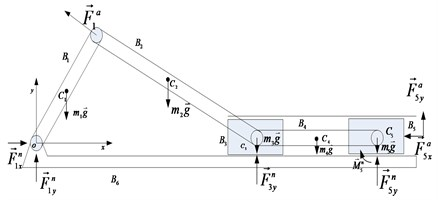From the second law of rigid body Newton, we get the differential equation of motion of centroid $c$:

7
$\left\{\begin{array}{c}m{\stackrel{¨}{x}}_{c}+{k}_{11}{x}_{D}+{k}_{12}{\theta }_{yc}={F}_{x},\\ m{\stackrel{¨}{y}}_{c}+{k}_{21}{y}_{D}+{k}_{22}{\theta }_{xc}={F}_{y},\end{array}\right\$

where ${k}_{11}$, ${k}_{12}$, ${k}_{21}$, ${k}_{22}$ is the bending stiffness, ${F}_{x}$, ${F}_{y}$ is the electromagnetic force caused by the electromagnetic field. From the momentum theorem, the differential equation of centroid rotation is obtained:

8
$\left\{\begin{array}{c}{J}_{d}\stackrel{¨}{{\theta }_{x}}+L\stackrel{˙}{{\theta }_{y}}-{k}_{32}y+{k}_{33}{\theta }_{x}=0,\\ {J}_{d}\stackrel{¨}{{\theta }_{y}}-L\stackrel{˙}{{\theta }_{x}}+{k}_{41}x+{k}_{44}{\theta }_{y}=0,\end{array}\right\$

where${k}_{32}$, ${k}_{33}$, ${k}_{41}$, ${k}_{44}$ for the axis bending stiffness. The relationship between the coordinates ${x}_{D}$, ${y}_{D}$ of the point $D$ in Fig. 3 and the coordinates of the two ends is:

9
$\left[\begin{array}{c}{x}_{D}\\ {\theta }_{y}\\ {y}_{D}\\ {\theta }_{x}\end{array}\right]=\left[\begin{array}{ccc}b/l& a/l& \begin{array}{cc}& \end{array}\\ -1/l& 1/l& \begin{array}{cc}& \end{array}\\ \begin{array}{c}\\ \end{array}& \begin{array}{c}\\ \end{array}& \begin{array}{cc}\begin{array}{c}b/l\\ -1/l\end{array}& \begin{array}{c}a/l\\ 1/l\end{array}\end{array}\end{array}\right]\left[\begin{array}{c}{x}_{1}\\ {x}_{2}\\ {y}_{1}\\ {y}_{2}\end{array}\right].$

Let the eccentricity of the disc be $e$ the initial phase is $\phi$, the relationship between the coordinate ${x}_{D}$, ${y}_{D}$ and the center of mass $C$ is:

10
$\left\{\begin{array}{c}{x}_{c}={x}_{D}+ecos\left(\mathrm{\Omega }t+\phi \right),\\ {y}_{c}={y}_{D}+esin\left(\mathrm{\Omega }t+\phi \right).\end{array}\right\$

Substituting Eq. (10) into Eq. (7):

11
$\left\{\begin{array}{c}m{\stackrel{¨}{x}}_{D}+{k}_{11}{x}_{D}+{k}_{12}{\theta }_{yc}={F}_{x}+me{\mathrm{\Omega }}^{2}cos\left(\mathrm{\Omega }t+\phi \right),\\ m{\stackrel{¨}{y}}_{D}+{k}_{21}{y}_{D}+{k}_{22}{\theta }_{xc}={F}_{y}+me{\mathrm{\Omega }}^{2}sin\left(\mathrm{\Omega }t+\phi \right).\end{array}\right\$

Eqs. (3)-(11) are the differential equations and boundary conditions of the motor rotor steady-state motion. From Fig. 4, the crankshaft, connecting rod and slider as a whole, by the base, crank, connecting rod, slider, guide rod and piston composed of six rigid body, and the centroid ${C}_{i}$ of the conjoined group is established. The vector diameters of the connected base points ${C}_{i}$ are ${\stackrel{\to }{e}}^{i}={\left({\stackrel{\to }{x}}^{i},{\stackrel{\to }{y}}^{i}\right)}^{T}$. The angle between the base vector ${\stackrel{\to }{x}}^{i}$ and the base vector ${\stackrel{\to }{x}}^{r}$ of the reference base is the attitude angle ${\phi }_{i}$ of the rigid body. The vector of the rigid body ${B}_{i}$ is formed by the vector ${\stackrel{\to }{r}}_{i}$ and the posture angle ${\phi }_{i}$ of each rigid body, and the coordinate array of the rigid body ${B}_{i}$ is:

12
${q}_{i}={\left({\stackrel{\to }{r}}_{i}^{T}{,\phi }_{i}\right)}^{T}={\left({x}_{i}{,y}_{i}{,\phi }_{i}\right)}^{T},\left(i=1,2,\dots ,6\right).$

The coordinate array of the system consists of 18 coordinates:

13
$q={\left({q}_{1}^{T}{,q}_{2}^{T}\cdots {q}_{6}^{T}\right)}^{T}={\left({x}_{1}{,y}_{1}{,\phi }_{1},{x}_{2}{,y}_{2},{\phi }_{2},\cdots {x}_{6}{,y}_{6}{,\phi }_{6}\right)}^{T}.$

The system has a total of four hinges (${H}_{1}~{H}_{5}$) and two sliding hinges (${H}_{6}~{H}_{7}$). Due to the slider ${B}_{5}$, the guide rod ${B}_{4}$ is in the same form as the slider ${B}_{3}$, thus adding two constraints. The total number of system constrained equations is 17, and the degree of freedom $\delta =$ 18-17 = 1. As the crank ${B}_{1}$ by the worm, to a certain angular velocity $\stackrel{\to }{\omega }$ movement, for the additional drive constraints, so the system for the whole system. In the system, the dots on the base ${B}_{6}$ and the crankshaft ${B}_{1}$ are constrained by the rotation hinges, as shown in Fig. 5. Let the coordinates of the point on the conjoined body ${\stackrel{\to }{e}}^{1}$ is ${\left(-{a}_{1},0\right)}^{T}$.

Is the direction cosine matrix of the conjoined base ${\stackrel{\to }{e}}^{1}$ relative to the reference coordinate base ${\stackrel{\to }{e}}^{r}$:

14
${A}^{r1}=\left[\begin{array}{cc}\mathrm{c}\mathrm{o}\mathrm{s}{\phi }_{1}& -\mathrm{s}\mathrm{i}\mathrm{n}{\phi }_{1}\\ \mathrm{s}\mathrm{i}\mathrm{n}{\phi }_{1}& \mathrm{c}\mathrm{o}\mathrm{s}{\phi }_{1}\end{array}\right].$

The coordinates of the crank ${B}_{1}$ on the reference coordinate base ${\stackrel{\to }{e}}^{r}$ are:

15
${\stackrel{\to }{r}}_{O}={\stackrel{\to }{r}}_{1}+{A}^{r1}{\stackrel{\to }{\rho }}_{O}^{1}.$

The coordinate relationship is explicit:

16
$\left\{\begin{array}{c}{x}_{O}^{r}\\ {y}_{O}^{r}\end{array}\right\}=\left\{\begin{array}{c}{x}_{1}\\ {y}_{1}\end{array}\right\}+\left[\begin{array}{cc}\mathrm{c}\mathrm{o}\mathrm{s}{\phi }_{1}& -\mathrm{s}\mathrm{i}\mathrm{n}{\phi }_{1}\\ \mathrm{s}\mathrm{i}\mathrm{n}{\phi }_{1}& \mathrm{c}\mathrm{o}\mathrm{s}{\phi }_{1}\end{array}\right]\left\{\begin{array}{c}-{a}_{1}\\ 0\end{array}\right\}=\left\{\begin{array}{c}{x}_{1}-{a}_{1}cos{\phi }_{1}\\ {y}_{1}-{a}_{1}sin{\phi }_{1}\end{array}\right\}.$

Thus the ${H}_{1}$ constraint equation is obtained:

17
$\left\{\begin{array}{c}{x}_{1}-{a}_{1}cos{\phi }_{1}\\ {y}_{1}-{a}_{1}sin{\phi }_{1}\end{array}\right\}=\left\{\begin{array}{c}0\\ 0\end{array}\right\}.$

In the same way, we can get the constrain equation of ${H}_{2}$~${H}_{8}$.From the function $\mathrm{\Phi }\left(q,t\right)$, the acceleration constraint equation is:

18
${\mathrm{\Phi }}_{q}\left(q,t\right)\stackrel{¨}{q}=-{\left({\mathrm{\Phi }}_{q}\stackrel{˙}{q}\right)}_{q}\stackrel{˙}{q}-2{\mathrm{\Phi }}_{qt}\stackrel{˙}{q}-{\mathrm{\Phi }}_{tt}.$

Fig. 5The base and crank restraints diagram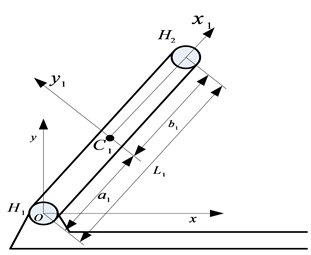Fig. 6The crank – slider mechanical model diagram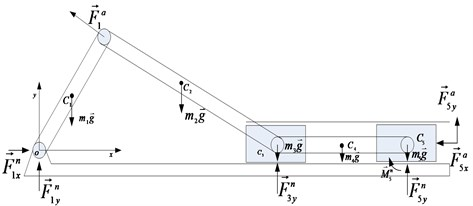### 2.2. Research on the dynamic characteristics of crank – slider mechanism

Bilge pump crank – slider mechanism, including slider, connecting rod, crankshaft and other three main components. Crank movement of the slider mechanism generated by the interference force transmitted through the bearing to the body, causing the pump vibration, so the purpose of the study is to grasp the bearing on the crankshaft of the reaction force. The components are used as rigid bodies, both ends of the rolling bearing is simplified as a hinge, and the crank-slider movement is simplified as a plane motion .

First, the crank-slider mechanical system as the object, the force shown in Fig. 6, the main force of the system, including the worm on the worm force ${\stackrel{\to }{F}}_{1}^{a}$, the hydraulic pressure on the piston force ${\stackrel{\to }{F}}_{5}^{a}$, the Rigid body mass ${m}_{i}\stackrel{\to }{g}$, rigid body motion process inertia force ${m}_{i}\stackrel{\to }{{a}_{i}}$. The binding force includes the force of the bearing on the crankshaft ${\stackrel{\to }{F}}_{1}^{n}$, the force of the body on the slider ${\stackrel{\to }{F}}_{3}^{n}$, the body of the piston force ${\stackrel{\to }{F}}_{5}^{n}$ and force even ${\stackrel{\to }{M}}_{5}^{n}$.

The crank and the slider are connected by a connecting rod, and the connecting rod can be simplified as a two-pole structure. The force of the worm on the worm wheel ${\stackrel{\to }{F}}_{1}^{a}$ along the tangential direction of the crank, the connecting rod on the crank force ${\stackrel{\to }{F}}_{1}^{c}$ along the connecting rod direction. The momentum theorem and the momentum moment of the moving point are applied to the crank centroid ${C}_{1}$ to obtain the crank kinetic equation:

19
$\left\{\begin{array}{c}{m}_{1}{\stackrel{¨}{x}}_{1}={F}_{1x}^{c}-{F}_{1x}^{a}+{F}_{1x}^{n},\\ {m}_{1}{\stackrel{¨}{y}}_{1}=-{F}_{1y}^{c}+{F}_{1y}^{a}+{F}_{1y}^{n}-{m}_{1}g,\\ {J}_{1}{\stackrel{¨}{\phi }}_{1}={\stackrel{\to }{M}}_{1}^{a}\left({\stackrel{\to }{F}}_{1}^{a}\right)+{\stackrel{\to }{M}}_{1}^{c}\left({\stackrel{\to }{F}}_{1}^{c}\right)+{\stackrel{\to }{M}}_{1}^{n}\left({\stackrel{\to }{F}}_{1}^{n}\right),\end{array}\right\$

as:

20
${Z}_{1}=\left[\begin{array}{ccc}{m}_{1}& & \\ & {m}_{1}& \\ & & {J}_{1}\end{array}\right],{q}_{1}=\left\{\begin{array}{c}{x}_{1}\\ {y}_{1}\\ {\phi }_{1}\end{array}\right\},{\stackrel{^}{F}}_{1}^{a}=\left\{\begin{array}{c}{F}_{1x}^{a}\\ {F}_{1y}^{a}\\ {M}_{1}^{a}\end{array}\right\},{\stackrel{^}{F}}_{1}^{n}=\left\{\begin{array}{c}{F}_{1x}^{n}\\ {F}_{1y}^{n}\\ {M}_{1}^{n}\end{array}\right\},{\stackrel{^}{F}}_{1}^{C}=\left\{\begin{array}{c}{F}_{1x}^{C}\\ {F}_{1y}^{C}\\ {M}_{1}^{C}\end{array}\right\}.$

The Eq. (21) is written in the form of a matrix:

21
${Z}_{1}{\stackrel{¨}{q}}_{1}＝{\stackrel{^}{F}}_{1}^{a}+{\stackrel{^}{F}}_{1}^{n}+{\stackrel{^}{F}}_{1}^{C}.$

## 3. Conclusions

1) Considering the influence of the moment of inertia of the rotor and the shear deformation of the shaft on the dynamic characteristics of the motor armature, the kinematic and kinetic differential equations of the armature rotor can be established by using the Timoshenko beam-axis model.

2) By moving the moving parts as rigid body, the two ends of the rolling bearing are simplified for the hinge, and the crank-slider movement is simplified to the plane movement. The seven parts of the rolling bearing, front and rear ends, cranks, connecting rod journals, worm gears and so on are modeled. The dynamics of the mechanism and the constraints are in line with the actual situation.